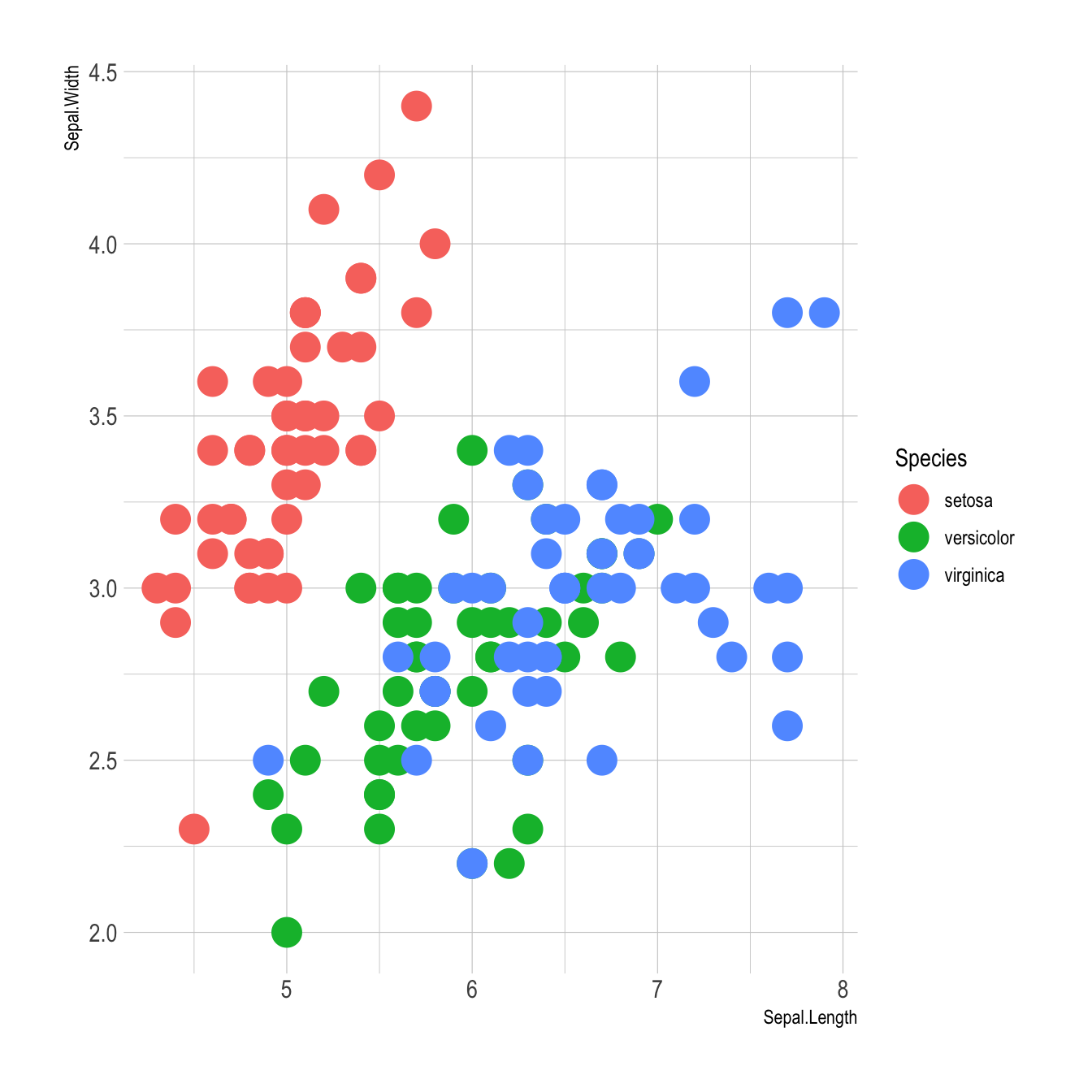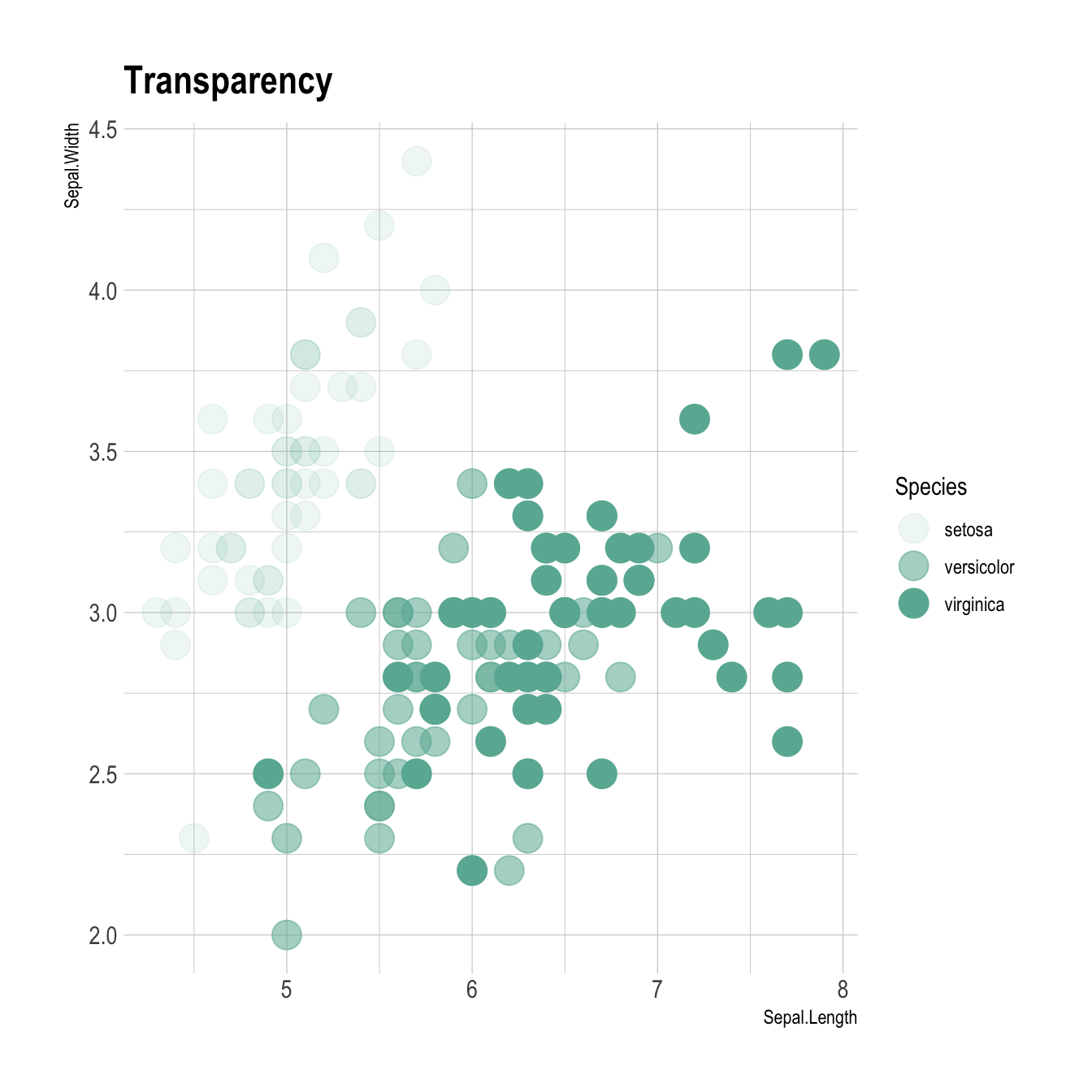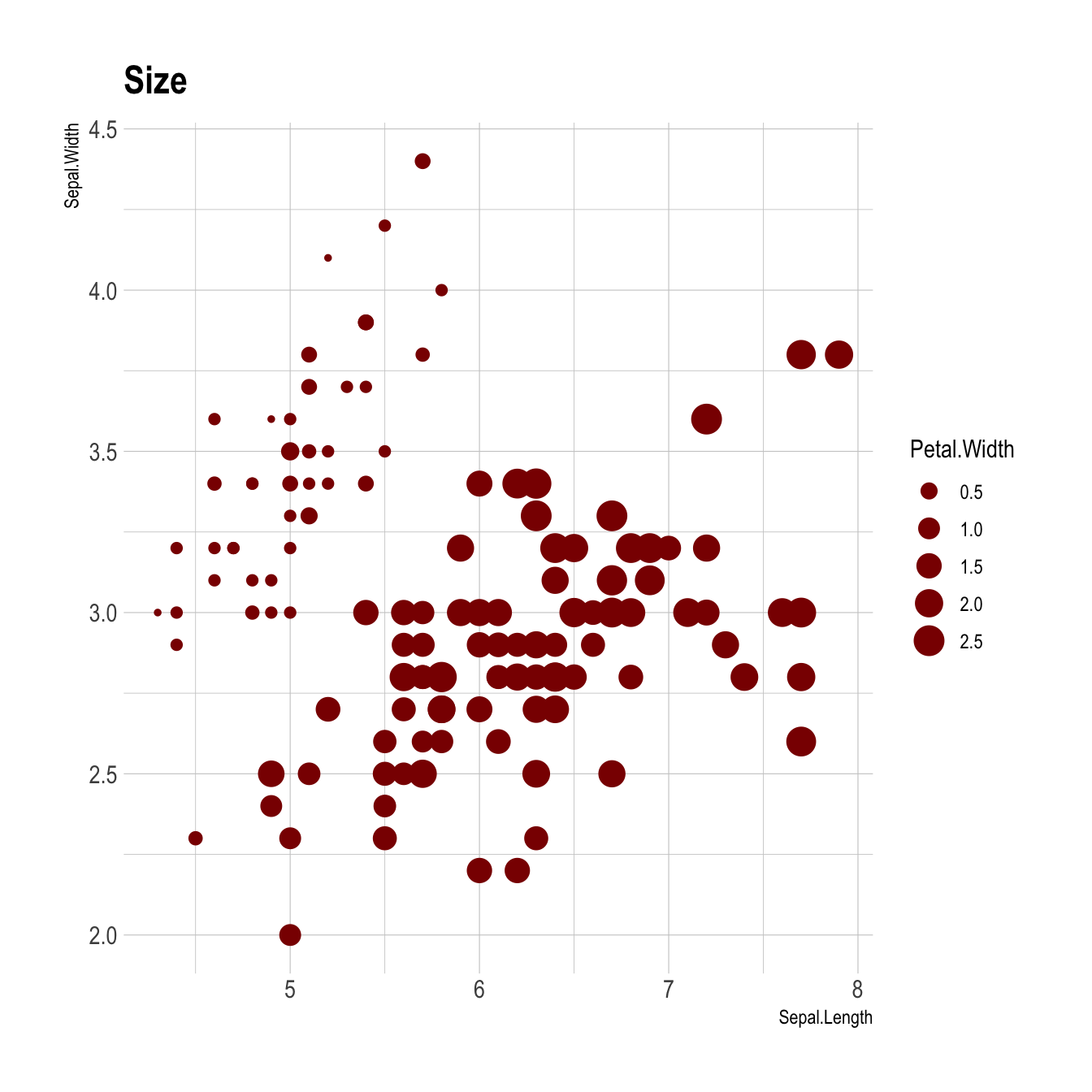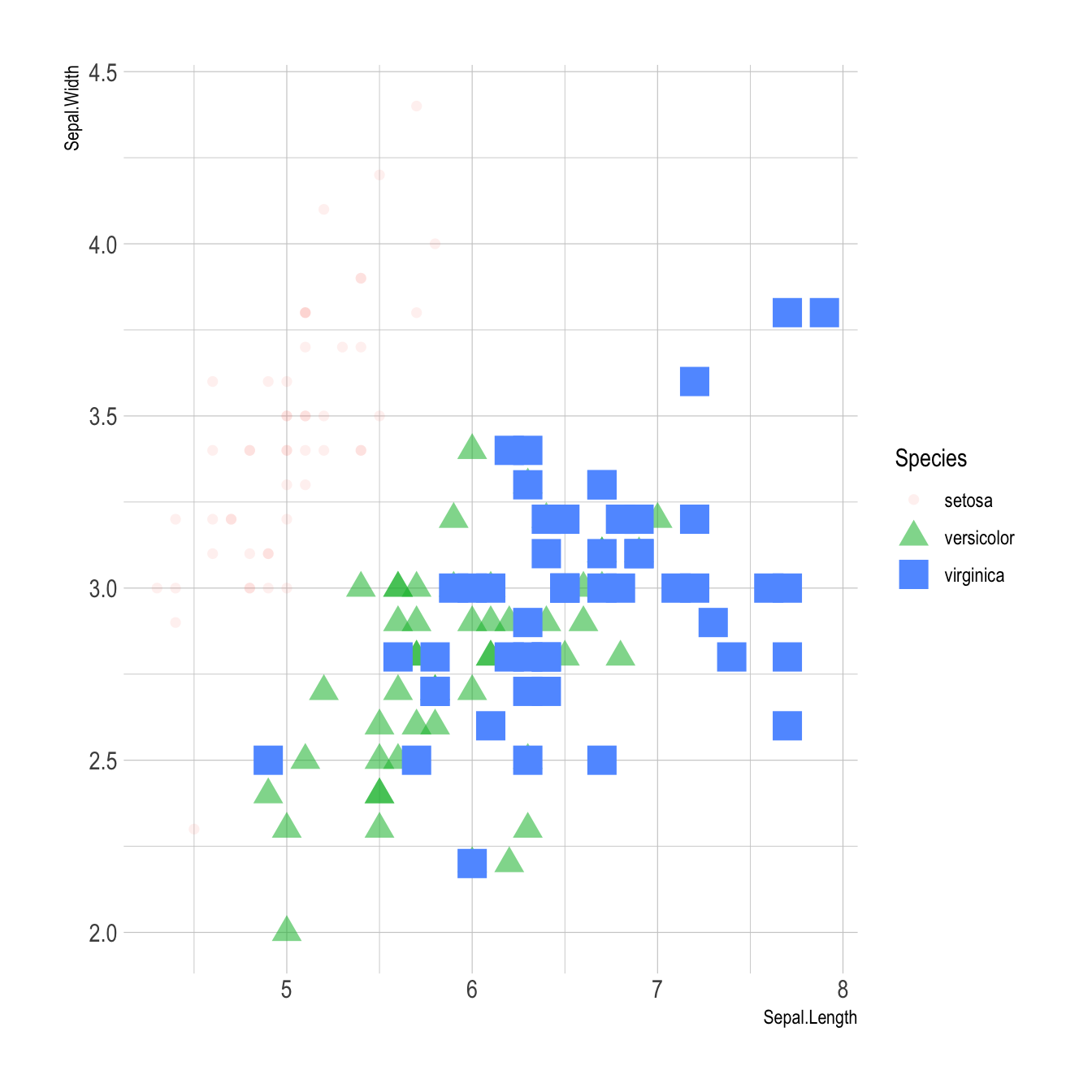# Map a variable to marker feature in ggplot2 scatterplot

`ggplot2` allows to easily map a variable to marker features of a scatterplot. This post explaines how it works through several examples, with explanation and code.

# Basic example

Here is the magick of ggplot2: the ability to map a variable to marker features. Here, the marker `color` depends on its value in the field called `Species` in the input data frame.

Note that the legend is built automatically.``````# load ggplot2
library(ggplot2)
library(hrbrthemes)

# mtcars dataset is natively available in R
# head(mtcars)

# A basic scatterplot with color depending on Species
ggplot(iris, aes(x=Sepal.Length, y=Sepal.Width, color=Species)) +
geom_point(size=6) +
theme_ipsum()``````

# Works with any aesthetics

You can map variables to any marker features. For instance, specie is represente below using transparency (left), shape (middle) and size (right).

``````# load ggplot2
library(ggplot2)
library(hrbrthemes)

# Transparency
ggplot(iris, aes(x=Sepal.Length, y=Sepal.Width, alpha=Species)) +
geom_point(size=6, color="#69b3a2") +
theme_ipsum()

# Shape
ggplot(iris, aes(x=Sepal.Length, y=Sepal.Width, shape=Species)) +
geom_point(size=6) +
theme_ipsum()

# Size
ggplot(iris, aes(x=Sepal.Length, y=Sepal.Width, shape=Species)) +
geom_point(size=6) +
theme_ipsum()``````# Mapping to several features

Last but not least, note that you can map one or several variables to one or several features. Here, shape, transparency, size and color all depends on the marker `Species` value.``````# load ggplot2
library(ggplot2)
library(hrbrthemes)

# A basic scatterplot with color depending on Species
ggplot(iris, aes(x=Sepal.Length, y=Sepal.Width, shape=Species, alpha=Species, size=Species, color=Species)) +
geom_point() +
theme_ipsum()``````

Related chart types

## Contact

This document is a work by Yan Holtz. Any feedback is highly encouraged. You can fill an issue on Github, drop me a message on Twitter, or send an email pasting yan.holtz.data with gmail.com.